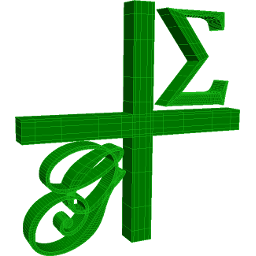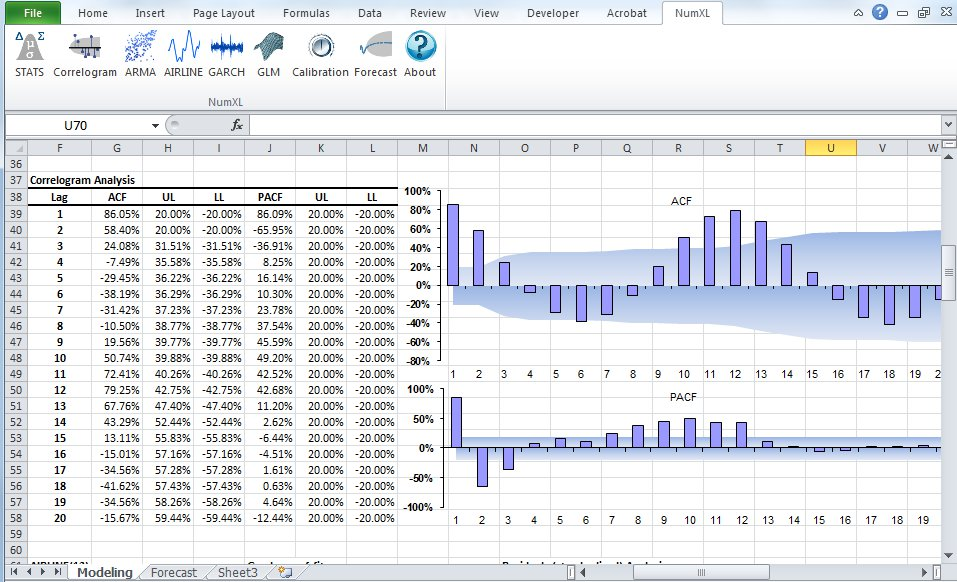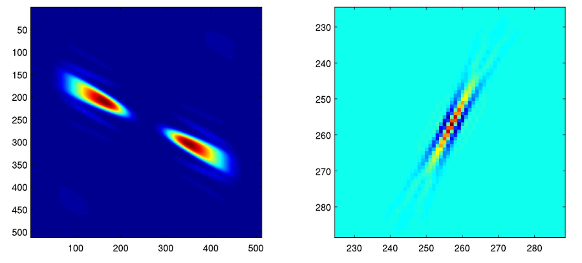## 34 Search Results

The package ADOL-C facilitates the evaluation of first and higher derivatives of vector functions that are defined by computer programs written in C or C++. The resulting derivative evaluation routines may be called from C/C++, Fortran, or any other language that can be linked with C. The numerical values of derivative vectors are obtained free of truncation errors at a small multiple of the run time and randomly accessed memory of the given function evaluation program.

### ATLAS

The ATLAS (Automatically Tuned Linear Algebra Software) project is an ongoing research effort focusing on applying empirical techniques in order to provide portable performance. At present, it provides C and Fortran77 interfaces to a portably efficient BLAS implementation, as well as a few routines from LAPACK.

### CGAL

CGAL is a collaborative effort of several sites in Europe and Israel. The goal is to make the most important of the solutions and methods developed in computational geometry available to users in industry and academia in a C++ library. The goal is to provide easy access to useful, reliable geometric algorithms The CGAL library contains: the Kernel with geometric primitives such as points, vectors, lines, predicates for testing things such as relative positions of points, and operations such as intersections and distance calculation, the Basic Library which is a collection of standard data structures and geometric algorithms, such as convex hull in 2D/3D, (Delaunay) triangulation in 2D/3D, planar map, polyhedron, smallest enclosing circle, and multidimensional query structures, the Support Library which offers interfaces to other packages, e.g., for visualisation, and I/O, and other support facilities.

### Convex

Convex is a Maple package for convex geometry. It can deal with rational polytopes, cones and other polyhedra of arbitrary dimension. Polyhedral complexes and fans are also supported, as well as computations in face lattices. The integration into Maple makes Convex particularly suited for "applied" problems where polyhedra arise together with other mathematical structures.

### Fast Artificial Neural Network Library

Fast Artificial Neural Network Library is a neural network library that implements multilayer artificial neural networks in C with support for both fully connected and sparsely connected networks. Cross-platform execution in both fixed and floating point are supported. It includes a framework for easy handling of training data sets. It is easy to use, versatile, well documented, and fast. PHP, C++, .NET, Python, Delphi, Octave, Ruby, Pure Data, and Mathematica bindings are available. A reference manual accompanies the library with examples and recommendations on how to use the library. A graphical user interface is also available for the library.

### FLINT: Fast Library for Number Theory

FLINT is a C library for doing number theory.

### G+Smo

G+Smo (Geometry + Simulation Modules, pronounced "gismo") is a new open-source C++ library that brings together mathematical tools for geometric design and numerical simulation. It is developed mainly by researchers and PhD students. It implements the relatively new paradigm of isogeometric analysis, which suggests the use of a unified framework in the design and analysis pipeline. G+Smo is an object-oriented, cross-platform, template C++ library and follows the generic programming principle, with a focus on both efficiency and ease of use. The library is partitioned into smaller entities, called modules. Examples of available modules include the dimension-independent NURBS module, the data fitting and solid segmentation module, the PDE discretization module and the adaptive spline module, based on hierarchical splines of arbitrary dimension and polynomial degree. The library is licenced under the Mozilla Public License v2.0. It has been developed within the homonym research network supported by the Austrian Science Fund and aims at providing access to high quality, open-source software to the forming isogeometric numerical simulation community and beyond.

More information### GELDA

GELDA is a Fortran77 software package for the numerical integration of general linear differential-algebraic equations (DAE) with variable coefficients of arbitrary index. The implementation of GELDA is based on the construction of the discretization scheme, which first determines all the local invariants and then transforms the linear DAE into an equivalent strangeness-free DAE with the same solution set. The resulting strangeness-free system is allowed to have nonuniqueness in the solution set or inconsistency in the initial values or inhomogeneities. In the case that the DAE is found to be uniquely solvable, GELDA is able to compute a consistent initial value and apply the well-known integration schemes for DAEs. In GELDA the BDF methods and Runge-Kutta schemes are implemented.

### GEOMS

GEOMS is a Fortran77 software package for the numerical integration of general model equations (equations of motion) of multibody systems. The code combines a regularization technique with the Runge-Kutta method of type Radau IIa of order 5. This regularization technique reduces the index under preservation of all information of the solution manifold and, therefore, allows a robust numerical integration of the system.

### Givaro

In the joint CNRS-INRIA / INPG-UJF project APACHE, Givaro is a C++ library for arithmetic and algebraic computations. Its main features are implementations of the basic arithmetic of many mathematical entities: Primes fields, Extensions Fields, Finite Fields, Finite Rings, Polynomials, Algebraic numbers, Arbitrary precision integers and rationals (C++ wrappers over gmp) It also provides data-structures and templated classes for the manipulation of basic algebraic objects, such as vectors, matrices (dense, sparse, structured), univariate polynomials (and therefore recursive multivariate). It contains different program modules and is fully compatible with the LinBox linear algebra library and the Athapascan environment, which permits parallel programming.

### Global Optimization Toolbox For Maple

Optimization is the science of finding solutions that satisfy complicated constraints and objectives. In engineering, constraints may arise from technical issues. In business, constraints are related to many factors, including cost, time, and staff. The objective of global optimization is to find [numerically] the absolute best solution of highly nonlinear optimization models that may have a number of locally optimal solutions. Global optimization problems can be extremely difficult. Frequently engineers and researchers are forced to settle for solutions that are “good enough” at the expense of extra time, money, and resources, because the best solution has not been found. Using the Global Optimization Toolbox, you can formulate your optimization model easily inside the powerful Maple numeric and symbolic system, and then use world-class Maple numeric solvers to return the best answer, fast! Illustrative references: 1. Pintér, J. D. Global Optimization in Action. Springer Science, 1996, 512 p., ISBN: 978-0-7923-3757-7 Winner of the 2000 INFORMS Computing Society Prize. 2. Pintér, J. D., Linder, D. and Chin, P. Global Optimization Toolbox for Maple: An introduction with illustrative applications. Optimization Methods and Software 21 (2006) (4) 565-582.

### GOBLIN Graph Library

A C++ class library including the whole bunch of standard algorithms in graph optimization and drawing. On top of this, a Tcl/Tk wrapper and a GUI for manipulating and editing of graphs.

### HSL

HSL (formerly the Harwell Subroutine Library) is a collection of ISO Fortran codes for large scale scientific computation, written by members of the Numerical Analysis Group and other experts.

### igraph

igraph is a collection of network analysis tools with the emphasis on efficiency, portability and ease of use. igraph is a free and open source software package for creating and manipulating undirected and directed graphs. It includes implementations for classic graph theory problems like minimum spanning trees and network flow, and also implements algorithms for some recent network analysis methods, like community structure search. The efficient implementation of igraph allows it to handle graphs with millions of vertices and edges. The rule of thumb is that if your graph fits into the physical memory then igraph can handle it. igraph can be programmed in R, Python and C/C++ by virtue of R/igraph, python-igraph and C/igraph, respectively. There is also a Mathematica interface IGraph/M written by Szabolcs Horvát.

### IML - Integer Matrix Library

IML is a free library of C source code which implements algorithms for computing exact solutions to dense systems of linear equations over the integers. IML is designed to be used with the ATLAS/BLAS library and GMP bignum library. Written in portable C, IML can be used on both 32-bit and 64-bit machines. It can be called from C++.

### Kenzo

Kenzo is a program for effective algebraic topology

### LiDIA

LiDIA is a C++ library for computational number theory which provides a collection of highly optimized implementations of various multiprecision data types and time-intensive algorithms.

### LinBox

LinBox is a C++ template library for exact, high-performance linear algebra computation with dense, sparse, and structured matrices over the integers and over finite fields. LinBox has the following top-level functions: solve linear system, matrix rank, determinant, minimal polynomial, characteristic polynomial, Smith normal form and trace. A good collection of finite field and ring implementations is provided, for use with numerous black box matrix storage schemes.

### Mastrave

Mastrave is a free software library written to perform vectorized scientific computing and to be as compatible as possible with both GNU Octave and Matlab computing frameworks, offering general purpose, portable and freely available features for the scientific community. Mastrave is mostly oriented to ease complex modelling tasks such as those typically needed within environmental models, even when involving irregular and heterogeneous data series. The set of array-based semantic constraints provided by the library implements the standard semantic support for the Semantic Array Programming (SemAP) paradigm [http://mfkp.org/INRMM/tag/semap]. This support is meant to allow concise pieces of array-programming code to be immersed within a semantic network of array-concepts, without renouncing to extremely compact representations. Based on the mathematics of arrays, the semantics of the SemAP constraints is inherently portable. A rich set of semantic constraints is natively implemented in GNU Octave/MATLAB by the Mastrave modelling library and is easily accessed in other programming languages via multi-language array programming bridges (e.g. in Python and GNU R). For a simplified summary of some core concepts, you may read https://dx.doi.org/10.6084/m9.figshare.3472661

More information### NetworkX

NetworkX (NX) is a Python package for the creation, manipulation, and study of the structure, dynamics, and functions of complex networks.

### NTL

NTL is a high-performance, portable C++ library providing data structures and algorithms for manipulating signed, arbitrary length integers, and for vectors, matrices, and polynomials over the integers and over finite fields.

### NumXL

NumXL is a suite of time series Excel add-ins. It transforms your Microsoft Excel application into a first-class time series software and econometrics tool, offering the kind of statistical accuracy offered by the leading statistical packages. NumXL integrates natively with Excel, adding scores of econometric functions, a rich set of shortcuts, and intuitive user interfaces to guide you through the entire process.

More information### PLTMG

PLTMG is a package for solving elliptic partial differential equations in general regions of the plane. It is based on continuous piecewise linear triangular finite elements, and features adaptive local mesh refinement, multigraph iteration, and pseudo-arclength continuation options for parameter dependencies. It also provides options for solving several classes of optimal control and obstacle problems. The package includes an initial mesh generator and several graphics packages. Support for the Bank-Holst parallel adaptive meshing strategy is also provided. PLTMG is provided as Fortran (and a little C) source code, in both single and double precision versions. The code has interfaces to X-Windows, MPI, and Michael Holst's OpenGL image viewer SG. The X-Windows, MPI, and SG interfaces require libraries that are NOT provided as part of the PLTMG package.

### PolyBoRi

The core of PolyBoRi is a C++ library, which provides high-level data types for Boolean polynomials and monomials, exponent vectors, as well as for the underlying polynomial rings and subsets of the powerset of the Boolean variables. As a unique approach, binary decision diagrams are used as internal storage type for polynomial structures. On top of this C++-library we provide a Python interface. This allows parsing of complex polynomial systems, as well as sophisticated and extendable strategies for Gröbner base computation. PolyBoRi features a powerful reference implementation for Gröbner basis computation.

More information### rbMIT

The rbMIT © MIT software package implements in Matlab® all the general reduced basis algorithms. The rbMIT © MIT software package is intended to serve both (as Matlab® source) "Developers" — numerical analysts and computational tool-builders — who wish to further develop the methodology, and (as Matlab® "executables") "Users" — computational engineers and educators — who wish to rapidly apply the methodology to new applications. The rbMIT software package was awarded with the Springer Computational Science and Engineering Prize in 2009.

### RCWA

RCWA is a package for the computer algebra system GAP. It provides implementations of algorithms and methods for computing in certain infinite permutation groups. The class of groups which RCWA in principle can deal with includes the finite groups, the free groups of finite rank, the free products of finitely many finite groups, certain infinite simple groups, certain divisible torsion groups and groups of many further types. It is closed under taking direct products and under taking wreath products with finite groups and with the infinite cyclic group (Z,+).

### Scilab Image Processing Toolbox

SIP provides image processing, pattern recognition, and computer vision routines for SciLab, a Matlab-like matrix-oriented programming environment. SIP is able to read/write images in almost 90 major formats, including JPEG, PNG, BMP, GIF, FITS, and TIFF. It includes routines for filtering, segmentation, edge detection, morphology, curvature, fractal dimension, distance transforms, multiscale skeletons, and more.

### ShearLab 3D

ShearLab 3D is a MATLAB Library, developed for processing two- and three-dimensional signals using a certain class of basis functions named shearlets. These functions were developed with the goal of constructing systems of basis-functions nicely suited for representing anisotropic features (e.g. curvilinear singularities) that often are present in multivariate data. ShearLab 3D is optimized for GPU computing with CUDA and contains examples for applications like image and video denoising or image and video inpainting.

More information### Steenrod

Steenrod is a Maple package for doing computations in the mod 2 Steenrod algebra. It computes the product and coproduct of elements, converts between various bases, computes the action of the elements on polynomials, and does several other specialized calculations related to the mod 2 Steenrod algebra.

### Sums over integral points of a polygon

Maple program for computing the sum of values of a polynomial function over the set of integral points of a polygon and the corresponding weighted Ehrhart quasi-polynomial.

### SuperLU

SuperLU is a general purpose library for the direct solution of large, sparse, nonsymmetric systems of linear equations on high performance machines. The library is written in C and is callable from either C or Fortran. The library routines will perform an LU decomposition with partial pivoting and triangular system solves through forward and back substitution. The LU factorization routines can handle non-square matrices but the triangular solves are performed only for square matrices. The matrix columns may be preordered (before factorization) either through library or user supplied routines. This preordering for sparsity is completely separate from the factorization. Working precision iterative refinement subroutines are provided for improved backward stability. Routines are also provided to equilibrate the system, estimate the condition number, calculate the relative backward error, and estimate error bounds for the refined solutions.

### SYMMETRICA

Symmetrica is a program developed by Lehrstuhl Mathematik II of the University of Bayreuth. It has routines to handle the following topics: ordinary representation theory of the symmetric group and related groups, ordinary representation theory of the classical groups, modular representation theory of the symmetric group, projective representation theory of the symmetric group, combinatorics of tableaux, symmetric functions and polynomials, commutative and non commutative Schubert polynomials, operations of finite groups, ordinary representation theory of Hecke algebras of type An